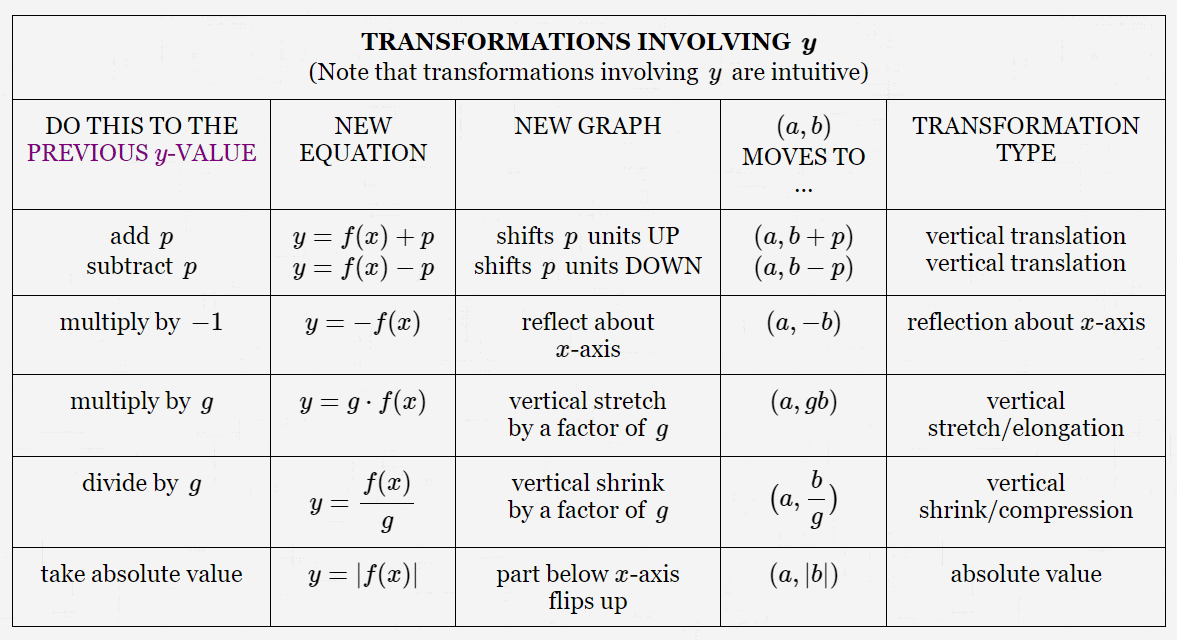﻿f What is the Graph of y = f(x)?

#What is the Graph of $\,y = f(x)\,$?

The lesson Graphs of Functions in the Algebra II curriculum gives a thorough introduction to graphs of functions.

For those who need only a quick review, the key concepts are repeated here. The exercises in this lesson duplicate (and then extend) those in Graphs of Functions.

• The equation ‘$\,y = f(x)\,$’ is an equation in two variables, $\,x\,$ and $\,y\,.$

The graph of the equation $\,y = f(x)\,$ is the picture of all the points $\,(x,y)\,$ that make it true. Observe that to make this equation true, $\,y\,$ must equal $\,f(x)\,.$

Thus, the graph of the equation $\,y = f(x)\,$ is the set of all points of the form $\,\color{green}{\bigl(x,\overbrace{f(x)}^{y}\bigr)}\,.$

• The graph of a function $\,f\,$ is the set of all its $\,\bigl(\text{input},\text{output}\bigr)\,$ pairs. Recall that when $\,x\,$ is an input, then $\,f(x)\,$ is the corresponding output.

Thus, the graph of $\,f\,$ is the set of all points of the form $\,\color{green}{\bigl(x,f(x)\bigr)}\,.$

• Compare! The following two requests ask for exactly the same thing:

Graph the equation $\,\color{green}{y = f(x)}\,.$

Graph the function $\,\color{green}{f}\,.$

Both are asking for the set of all points of the form $\,\bigl(x,f(x)\bigr)\,.$

## Understanding the Relationship Between an Equation and its Graph

There are things that you can DO to an equation $\,y = f(x)\,$ that will change its graph.

Or, there are things that you can DO to a graph that will change its equation.

Stretching, shrinking, moving up/down left/right, reflecting about axes—they're all covered thoroughly in the next few web exercises:

An understanding of these graphical transformations makes it easy to graph a wide variety of functions, by starting with a basic model and then applying a sequence of transformations to change it to the desired function.

For example, after mastering the graphical transformations, you'll be able to do the following:

• Need the graph of $\,y = -\sqrt{3x} + 2\,$? No problem!

Start with the graph of $\,y = \sqrt{x}\,$ (one of the basic models):

(A) Divide all the $x$-values by $\,3\,$ (a horizontal compression/shrink—the points get closer to the $y$-axis).

(B) Then reflect about the $x$-axis.

(C) Then move it up $\,2\,.$

You'll understand why the order BAC would also work, but the order CAB doesn't!

• Or, suppose you have the graph (call it $\,G\,$) of a known equation $\,y = f(x)\,.$ The graph is being changed, though, and you need the corresponding new equations.

If $\,G\,$ is shifted up $\,2\,$ units, the new equation is $\,y = f(x) + 2\,.$ (Transformations involving $\,y\,$ are intuitive.)

If $\,G\,$ is shifted right $\,2\,$ units, the new equation is $\,y = f(x-2)\,.$ (Transformations involving $\,x\,$ are counter-intuitive.)

If $\,G\,$ is reflected about the $y$-axis, the new equation is $\,y = f(-x)\,.$

For your convenience, all the graphical transformations are summarized in the Graphical Transformations tables below. Given any entry in a row, you should (eventually!) be able to fill in all the remaining entries in that row.

(Cases 18–24 below help you master the ideas in the Graphical Transformation tables!)

## Summary: Graphical Transformations

### Set-Up for the Tables:

• You're starting with the equation $\,y = f(x)\,.$ So, the ‘previous $\color{purple}{y}$-value (see the first column below) is $\,f(x)\,.$

(For a mobile-friendly design, the columns turned into rows. Images of the original table formatting are given below.)

• Assume:
$p \gt 0$    (‘$p$’ for Positive)
$g \gt 1$    (‘$g$’ for Greater than)
• The point $\,(a,b)\,$ is a point on the graph of $\,y = f(x)\,,$ so that the equation $\,b = f(a)\,$ is true.

Image of original table formatting:TRANSFORMATIONS INVOLVING $\,\boldsymbol{y}$ (Note that transformations involving $\,y\,$ are intuitive) Do This to the Previous $y$-value: add $\,p$subtract $\,p$ New Equation: $y = f(x) + p$$y = f(x) - p New Graph: shifts \,p\, units UPshifts \,p\, units DOWN (a,b) Moves to ... (a,b+p)$$(a,b-p)$ Transformation Type: vertical translationvertical translation
 TRANSFORMATIONS INVOLVING $\,\boldsymbol{y}$ (intuitive) Do This to the Previous $y$-value: multiply by $\,-1$ New Equation: $y = -f(x)$ New Graph: reflect about $x$-axis $(a,b)$ Moves to ... $(a,-b)$ Transformation Type: reflection about $x$-axis
 TRANSFORMATIONS INVOLVING $\,\boldsymbol{y}$ (intuitive) Do This to the Previous $y$-value: multiply by $\,g$ New Equation: $y = g\cdot f(x)$ New Graph: vertical stretch by a factor of $\,g$ $(a,b)$ Moves to ... $(a,gb)$ Transformation Type: vertical stretch/elongation
 TRANSFORMATIONS INVOLVING $\,\boldsymbol{y}$ (intuitive) Do This to the Previous $y$-value: divide by $\,g$ New Equation: $\displaystyle y = \frac{f(x)}{g}$ New Graph: vertical shrink by a factor of $\,g$ $(a,b)$ Moves to ... $\displaystyle \bigl(a,\frac{b}{g}\bigr)$ Transformation Type: vertical shrink/compression
 TRANSFORMATIONS INVOLVING $\,\boldsymbol{y}$ (intuitive) Do This to the Previous $y$-value: take absolute value New Equation: $y = |f(x)|$ New Graph: part below $x$-axis flips up $(a,b)$ Moves to ... $(a,|b|)$ Transformation Type: absolute value

Image of original table formatting:TRANSFORMATIONS INVOLVING $\,\boldsymbol{x}$ (Note that transformations involving $\,x\,$ are counter-intuitive) Replace ... every $\,x\,$ by $\,x+p$every $\,x\,$ by $\,x-p$ New Equation: $y = f(x+p)$$y = f(x-p) New Graph: shifts \,p\, units LEFT shifts \,p\, units RIGHT (a,b) Moves to ... (a-p,b)$$(a+p,b)$ Transformation Type: horizontal translationhorizontal translation
 TRANSFORMATIONS INVOLVING $\,\boldsymbol{x}$ (counter-intuitive) Replace ... every $\,x\,$ by $\,-x\,$ New Equation: $y = f(-x)$ New Graph: reflect about $y$-axis $(a,b)$ Moves to ... $(-a,b)$ Transformation Type: reflection about $y$-axis
 TRANSFORMATIONS INVOLVING $\,\boldsymbol{x}$ (counter-intuitive) Replace ... every $\,x\,$ by $\,gx\,$ New Equation: $y = f(gx)$ New Graph: horizontal shrinkby a factor of $\,g$ $(a,b)$ Moves to ... $\displaystyle \bigl(\frac{a}{g},b\bigr)$ Transformation Type: horizontal shrink/compression
 TRANSFORMATIONS INVOLVING $\,\boldsymbol{x}$ (counter-intuitive) Replace ... every $\,x\,$ by $\,\displaystyle \frac{x}{g}\,$ New Equation: $\displaystyle y = f\bigl(\frac{x}{g}\bigr)$ New Graph: horizontal stretchby a factor of $\,g$ $(a,b)$ Moves to ... $(ga,b)$ Transformation Type: horizontal stretch/elongation